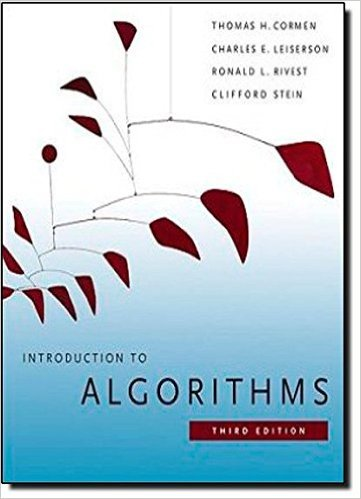×
Get Full Access to Introduction To Algorithms - 3 Edition - Chapter 15 - Problem 15-7
Get Full Access to Introduction To Algorithms - 3 Edition - Chapter 15 - Problem 15-7

×

# Viterbi algorithm We can use dynamic programming on aISBN: 9780262033848 130

## Solution for problem 15-7 Chapter 15

Introduction to Algorithms | 3rd Edition

• Textbook Solutions
• 2901 Step-by-step solutions solved by professors and subject experts
• Get 24/7 help from StudySoup virtual teaching assistantsIntroduction to Algorithms | 3rd Edition

4 5 1 387 Reviews
13
1
Problem 15-7

Viterbi algorithm We can use dynamic programming on a directed graph G D .V; E/ for speech recognition. Each edge .u; / 2 E is labeled with a sound .u; / from a fi- nite set of sounds. The labeled graph is a formal model of a person speaking for Chapter 15 409 a restricted language. Each path in the graph starting from a distinguished vertex 0 2 V corresponds to a possible sequence of sounds produced by the model. We define the label of a directed path to be the concatenation of the labels of the edges on that path. a. Describe an efficient algorithm that, given an edge-labeled graph G with distinguished vertex 0 and a sequence s D h 1; 2;:::; ki of sounds from , returns a path in G that begins at 0 and has s as its label, if any such path exists. Otherwise, the algorithm should return NO-SUCH-PATH. Analyze the running time of your algorithm. (Hint: You may find concepts from Chapter 22 useful.) Now, suppose that every edge .u; / 2 E has an associated nonnegative probability p.u; / of traversing the edge .u; / from vertex u and thus producing the corresponding sound. The sum of the probabilities of the edges leaving any vertex equals 1. The probability of a path is defined to be the product of the probabilities of its edges. We can view the probability of a path beginning at 0 as the probability that a random walk beginning at 0 will follow the specified path, where we randomly choose which edge to take leaving a vertex u according to the probabilities of the available edges leaving u. b. Extend your answer to part (a) so that if a path is returned, it is a most probable path starting at 0 and having label s. Analyze the running time of your algorithm. 15-8 Im

Step-by-Step Solution:
Step 1 of 3

L7 - 2 Def. One-Sided Limits We say that a functio fn(x)asm t L as x approaches the numberc from the right if we can make every value of f(x) as close to L as we want by choosing x suﬃciently close to c but x>c . We write thir sight-hand limit as lim f(x)= L. x→c + c y=f(x) c y=f(x) Similarly, the left-hand limit lx→cf−x)= L means we can make every value of f(x)asoeo L as we want by choosingx suﬃciently close to c but x 0 lim g(x)= l g(x)= x→0− x→0 + NOTE: lim f(x)= L if and only if x→c

Step 2 of 3

Step 3 of 3

##### ISBN: 9780262033848

Unlock Textbook Solution